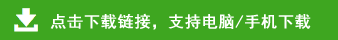# 三年级下册数学易错题及难题集锦

三年级下册数学易错题及难题集锦部分内容预览

填空
常用的面积单位有（    ）（      ）（      ）
1m2=（      ）dm       1dm2=(       ) cm
2m=（      ）dm        2dm2=(       ) cm
3m=（      ）dm        3dm2=(       ) cm
6m=（      ）dm         6dm=(       ) cm
100cm=(       ) dm    100dm=(       ) m
300cm=(       ) dm    200dm=(       ) m
16m=（      ）dm     9000cm=(       ) dm
12m=(      )dm=（       ）cm
6m=（       ）cm
3dm=(       ) cm
1500dm=(       ) m
一个茶杯高约13（       ）
一支铅笔约长16（      ）
我走一步约是30（         ）
一张邮票的面积约为6（   ）
小红手指甲盖面积约为1（     ）
数学作业本的面积约是4（  ）
数学作业本长18（      ）， 面积约4（       ）
一块正方形丝巾的面积400（    ）
北京颐和园的占地面积约为290000（           ）
一间教室的面积约是60（        ）
操场面积约10000（       ）
列竖式计算
39×11=          18×29=        37×64=      35×26=
脱式计算
37×49－1703      25×8×7       （136+72）÷2
280－100÷5        48×52÷2     18×27－320      78×59